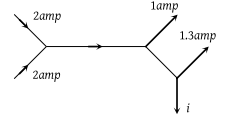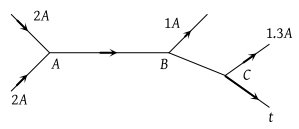# NEET Questions Solved

PMT - 1981

The figure below shows currents in a part of electric circuit. The current i is(1) 1.7 amp

(2) 3.7 amp

(3) 1.3 amp

(4) 1 amp

(1) According to Kirchhoff's first lawAt junction A, ${i}_{AB}=2+2=4A$

At junction B, ${i}_{AB}={i}_{BC}-1=3A$

At junction C, $i={i}_{BC}-1.3=3-1.3=1.7\text{\hspace{0.17em}}amp$

Difficulty Level:

• 72%
• 13%
• 11%
• 6%
Crack NEET with Online Course - Free Trial (Offer Valid Till September 17, 2019)